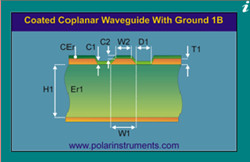## PCB Blog

### PCB impedance design and matchingPCB impedance matching refers to a suitable collocation between the signal source or the transmission line and the load. According to the access mode, there are two ways of impedance matching: serial and parallel. According to the frequency impedance matching of the signal source, it can be divided into two kinds: low frequency and high frequency. The impedance is directly proportional to the thickness of the dielectric and the line distance, inversely proportional to the linewidth, dielectric constant and copper thickness.

(1) high frequency signals are usually matched by serial impedance. The resistance value of the serial resistor is 20~75Ω. The resistance value is directly proportional to the signal frequency and inversely proportional to the PCB line width.In embedded systems, a serial matching resistor is required when the PCB line length is greater than 20M, and the line length is greater than 5cm, such as clock signals, data and address bus signals in the system.There are two functions of the serial matching resistor:

Reduce high frequency noise and edge overshoot. If the edge of a signal is very steep, it contains a large amount of high frequency components, which will cause radiation interference, and can also cause overshoot. A RC circuit is formed by the series resistance, the distribution of the signal line, the capacitance, and the load input capacitance, so that the steepness of the signal edge is reduced.

Reduce high frequency reflection and self-excited oscillation. When the frequency of the signal is very high, the wavelength of the signal is very short. When the wavelength is shorter than the length of the transmission line, the reflected signal superimposed on the original signal will change the shape of the original signal. If the characteristic impedance of the transmission line is not equal to the load impedance (i.e., mismatch), a reflection is generated at the load end, resulting in self-excited oscillations. the low frequency signal is directly connected to the inside of the PCB , and there is no need to add serial matching resistors.

(2) parallel impedance matching, also called terminal impedance matching, is usually used at the input / output interface, mainly refers to the impedance matching with the transmission cable. For example, LVDS and RS422/485 use 5 twisted pair inputs with a matching resistance of 100~120Ω; the video signal uses a coaxial cable with a matching resistance of 75Ω or 50Ω, using a flat cable of 300Ω. The resistance of the parallel matching resistor is related to the medium of the transmission cable. It is independent of the length, and its main function is to prevent the signal from reflecting and to reduce the self-excited oscillation.

It is worth mentioning that impedance matching can improve the EMI performance of the system. In addition, impedance matching can be done by using transformer instead of serial / parallel resistors. Typical examples, such as Ethernet interface and CAN bus, can be used in impedance matching.

Hits：    【PrintLiveChatclose